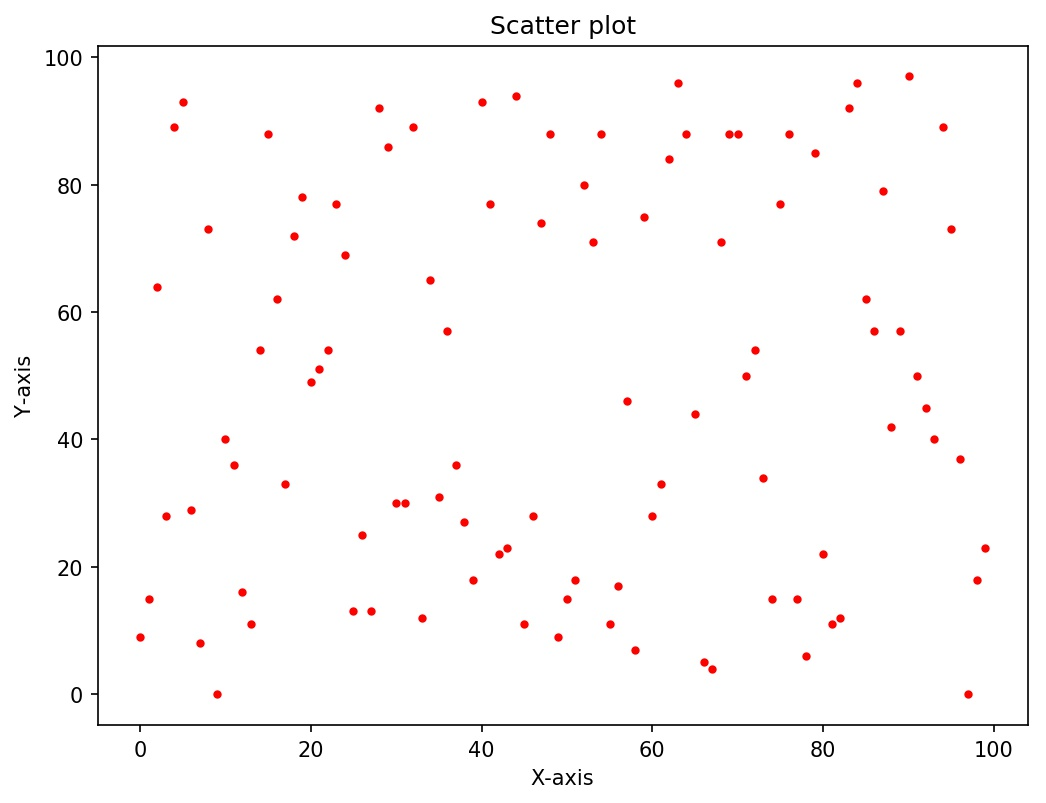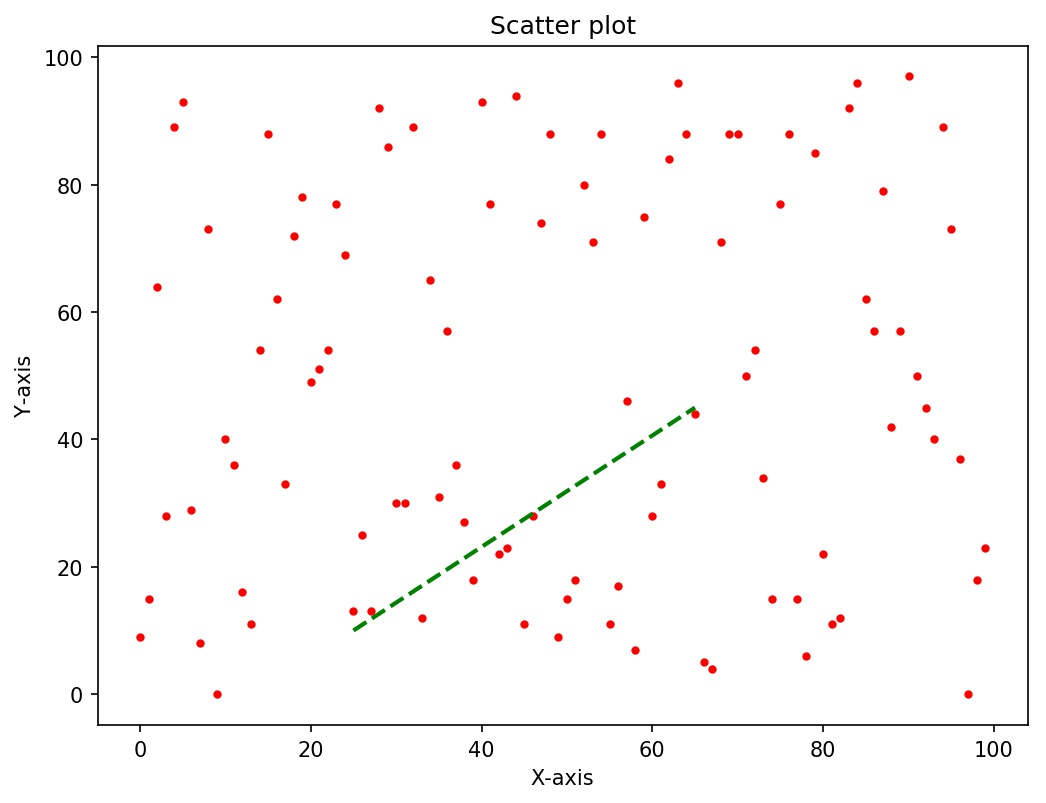# How to add an arbitrary line in a matplotlib plot in Python?

Hello Everyone!

In this tutorial, we are going to learn how to plot an arbitrary straight line in a matplotlib plot. As we know that a simple 2D plot contains two axes- X-axis and Y-axis. To plot a line, we need two points on the XY plane through which the line would be passing or connecting them. Therefore, it is necessary to choose some point (x1,y1) and (x2,y2) in order to draw the arbitrary line.

## Drawing an arbitrary line in a matplotlib plot

First of all, we would need a matplotlib on which we would be drawing the arbitrary line. Let us first plot a random scatter plot. Next, we would plot the line that would be bounded in the range: [x1,x2] and [y1,y2] or we can say connecting the two points (x1,y1) & (x2,y2).

Lets us take an example

Consider a random scatter plot below with the corresponding code.

```import numpy as np
import matplotlib.pyplot as plt
np.random.seed(10)
x = np.arange(0, 100)
y = np.random.randint(0, 100, 100) #random y points
fig = plt.figure(figsize=(8,6))  #setting the figure size
plt.scatter(x, y,marker='.',color='r') # plotting the scatter plot
plt.title('Scatter plot')
plt.xlabel('X-axis')
plt.ylabel('Y-axis')
plt.show()```

The output will show the plot given below:Now lets us draw an arbitrary line through this line. We can do this directly by providing the corresponding boundary range in the plot function of the matplotlib.

`plt.plot([X1, X2], [Y1, Y2],color='green',linewidth=2)`

We can also set the formatting options like color, line width, line style, marker style, marker width, etc.

#### Adding the arbitrary line to the scatter plot

Since our scatter plot is ready, we would add an arbitrary line to the plot. As an example, let us consider the boundary range [25,65] & [10,45].
Here x1=25, x2=65, y1=10, y2=45. Consider the code below which adds a dashed green line from point (25,10) to (65,45) to the above scatter plot. You can also add the marker if needed.

Code:

```import numpy as np
import matplotlib.pyplot as plt
np.random.seed(10)
x = np.arange(0, 100)
y = np.random.randint(0, 100, 100) #random y points
fig = plt.figure(figsize=(8,6))  #setting the figure size
plt.scatter(x, y,marker='.',color='r') # plotting the scatter plot
plt.title('Scatter plot')
plt.xlabel('X-axis')
plt.ylabel('Y-axis')
plt.plot([25, 65], [10, 45],color='green',
linestyle='dashed',linewidth=2)  # plotting the arbitrary line from point (25,10) to (65,45).
plt.show()```

Output:I hope you liked the article. Comment if you have any doubts or suggestions regarding this article.

You can also read other articles related to this. Click the links given below.

Save a Plot as an Image in Python

Line Chart Plotting in Python with Matplotlib